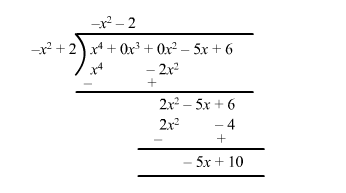# Find the quotient and remainder whenQuestion:

Find the quotient and remainder when

$f(x)=x^{4}-5 x+6$ is divided by $g(x)=2-x^{2}$

Solution:

We can write

$f(x)$ as $x^{4}+0 x^{3}+0 x^{2}-5 x+6$ and $g(x)$ as $-x^{2}+2$Quotient $q(x)=-x^{2}-2$

Remainder $r(x)=-5 x+10$